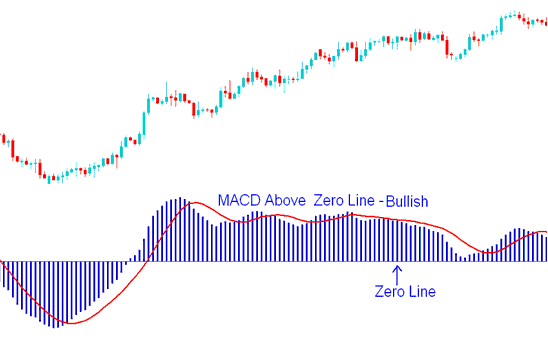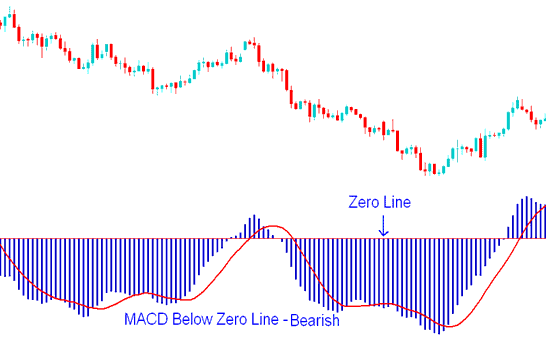# MACD Center Line Crossover

The MACD stock indices indicator is one of the most widely and commonly used indices indicators available. MACD indicator is a momentum oscillator with some indices trend following characteristics.

MACD is one of the most popular indicators used in stock indexes trading technical analysis. MACD indicator is used to generate stock indexes signals using crossovers.

MACD plots the divergence and convergence of moving averages. MACD indicator is constructed using moving average analysis. Moving Average Convergence/Divergence is a trend-following indices technical indicator. MACD indicator shows the correlation between two moving averages.

One moving average is of a shorter period and the other for a longer period of stock indexes price bars.MACD Stock Indexes Indicator - MACD Stock Indexes Indicator Technical Analysis

MACD stock indices indicator has a zero center line; values above zero line are bullish while those below zero are bearish.

In an upward indices trend the shorter MACD line rises faster that the longer MACD line this creates a gap. In addition as long as the MACD indicator is above the center mark the indices trend is still bullish as shown below.

Do not sell as long as the MACD Indices Indicator is above the Center Mark - this is bullish territory and it does not matter how it is moving as long as it is above the zero center mark, as shown on the stock indexes trading example explained and illustrated below.MACD Indices Indicator Above Zero Mark - Bullish Indices Trading Signal

In a downward indices trend the shorter MACD line falls faster than the longer MACD line this creates a gap. In addition as long as the indicator is below the center mark the indices trend is still bearish as shown below.

Do not buy as long as the MACD Indices Indicator is below the Center Mark - this is bearish territory and it does not matter how it is moving as long as it is below the zero center mark, as shown on the stock indexes trading example explained and illustrated below.MACD Indices Indicator Below Zero Center Line Mark - Bearish Indices Trading Signal

When the indices trend is about to reverse the MACD lines start to move closer to each other, thus closing the gap.